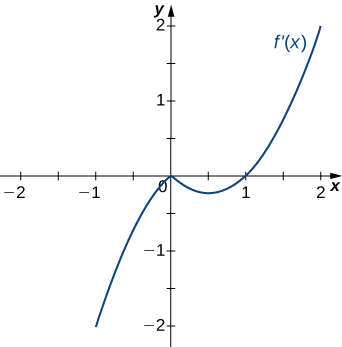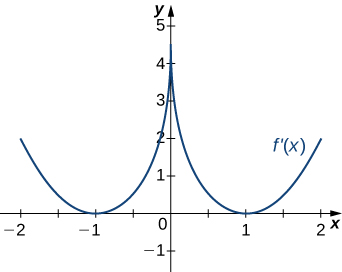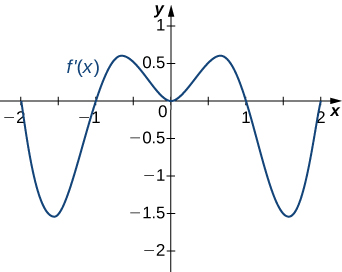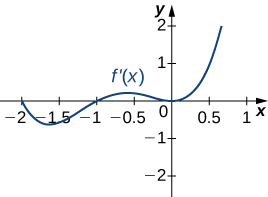# 4.4E: Exercises for Section 4.4

$$\newcommand{\vecs}{\overset { \rightharpoonup} {\mathbf{#1}} }$$ $$\newcommand{\vecd}{\overset{-\!-\!\rightharpoonup}{\vphantom{a}\smash {#1}}}$$$$\newcommand{\id}{\mathrm{id}}$$ $$\newcommand{\Span}{\mathrm{span}}$$ $$\newcommand{\kernel}{\mathrm{null}\,}$$ $$\newcommand{\range}{\mathrm{range}\,}$$ $$\newcommand{\RealPart}{\mathrm{Re}}$$ $$\newcommand{\ImaginaryPart}{\mathrm{Im}}$$ $$\newcommand{\Argument}{\mathrm{Arg}}$$ $$\newcommand{\norm}{\| #1 \|}$$ $$\newcommand{\inner}{\langle #1, #2 \rangle}$$ $$\newcommand{\Span}{\mathrm{span}}$$ $$\newcommand{\id}{\mathrm{id}}$$ $$\newcommand{\Span}{\mathrm{span}}$$ $$\newcommand{\kernel}{\mathrm{null}\,}$$ $$\newcommand{\range}{\mathrm{range}\,}$$ $$\newcommand{\RealPart}{\mathrm{Re}}$$ $$\newcommand{\ImaginaryPart}{\mathrm{Im}}$$ $$\newcommand{\Argument}{\mathrm{Arg}}$$ $$\newcommand{\norm}{\| #1 \|}$$ $$\newcommand{\inner}{\langle #1, #2 \rangle}$$ $$\newcommand{\Span}{\mathrm{span}}$$$$\newcommand{\AA}{\unicode[.8,0]{x212B}}$$

1) If $$c$$ is a critical point of $$f(x)$$, when is there no local maximum or minimum at $$c$$? Explain.

2) For the function $$y=x^3$$, is $$x=0$$ both an inflection point and a local maximum/minimum?

It is not a local maximum/minimum because $$f'$$ does not change sign

3) For the function $$y=x^3$$, is $$x=0$$ an inflection point?

4) Is it possible for a point $$c$$ to be both an inflection point and a local extremum of a twice differentiable function?

No

5) Why do you need continuity for the first derivative test? Come up with an example.

6) Explain whether a concave-down function has to cross $$y=0$$ for some value of $$x$$.

False; for example, $$y=\sqrt{x}$$.

7) Explain whether a polynomial of degree $$2$$ can have an inflection point.

In exercises 8 - 12, analyze the graphs of $$f'$$, then list all intervals where $$f$$ is increasing or decreasing.

8)Increasing for $$−2<x<−1$$ and $$x>2$$;
Decreasing for $$x<−2$$ and $$−1<x<2$$

9)10)Decreasing for $$x<1$$,
Increasing for $$x>1$$

11)12)Decreasing for $$−2<x<−1$$ and $$1<x<2$$;
Increasing for $$−1<x<1$$ and $$x<−2$$ and $$x>2$$

In exercises 13 - 17, analyze the graphs of $$f',$$ then list all intervals where

a. $$f$$ is increasing and decreasing and

b. the minima and maxima are located.

13)14)a. Increasing over $$−2<x<−1,\;0<x<1,x>2$$, Decreasing over $$x<−2, \;−1<x<0, \;1<x<2;$$
b. Maxima at $$x=−1$$ and $$x=1$$, Minima at $$x=−2$$ and $$x=0$$ and $$x=2$$

15)16)a. Increasing over $$x>0$$, Decreasing over $$x<0;$$
b. Minimum at $$x=0$$

17)In exercises 18 - 22, analyze the graphs of $$f'$$, then list all inflection points and intervals $$f$$ that are concave up and concave down.

18)Concave up for all $$x$$,
No inflection points

19)20)Concave up for all $$x$$,
No inflection points

21)22)Concave up for $$x<0$$ and $$x>1$$,
Concave down for $$0<x<1$$,
Inflection points at $$x=0$$ and $$x=1$$

For exercises 23 - 27, draw a graph that satisfies the given specifications for the domain $$x=[−3,3].$$ The function does not have to be continuous or differentiable.

23) $$f(x)>0,\;f'(x)>0$$ over $$x>1,\;−3<x<0,\;f'(x)=0$$ over $$0<x<1$$

24) $$f'(x)>0$$ over $$x>2,\;−3<x<−1,\;f'(x)<0$$ over $$−1<x<2,\;f''(x)<0$$ for all $$x$$

25) $$f''(x)<0$$ over $$−1<x<1,\;f''(x)>0,\;−3<x<−1,\;1<x<3,$$ local maximum at $$x=0,$$ local minima at $$x=±2$$

26) There is a local maximum at $$x=2,$$ local minimum at $$x=1,$$ and the graph is neither concave up nor concave down.

27) There are local maxima at $$x=±1,$$ the function is concave up for all $$x$$, and the function remains positive for all $$x.$$

For the following exercises, determine

a. intervals where $$f$$ is increasing or decreasing and

b. local minima and maxima of $$f$$.

28) $$f(x)=\sin x+\sin^3x$$ over $$−π<x<π$$

a. Increasing over $$−\frac{π}{2}<x<\frac{π}{2},$$ decreasing over $$x<−\frac{π}{2},\; x>\frac{π}{2}$$

b. Local maximum at $$x=\frac{π}{2}$$; local minimum at $$x=−\frac{π}{2}$$

29) $$f(x)=x^2+\cos x$$

For exercise 30, determine

a. intervals where $$f$$ is concave up or concave down, and

b. the inflection points of $$f$$.

30) $$f(x)=x^3−4x^2+x+2$$

a. Concave up for $$x>\frac{4}{3},$$ concave down for $$x<\frac{4}{3}$$

b. Inflection point at $$x=\frac{4}{3}$$

For exercises 31 - 37, determine

a. intervals where $$f$$ is increasing or decreasing,

b. local minima and maxima of $$f$$,

c. intervals where $$f$$ is concave up and concave down, and

d. the inflection points of $$f.$$

31) $$f(x)=x^2−6x$$

32) $$f(x)=x^3−6x^2$$

a. Increasing over $$x<0$$ and $$x>4,$$ decreasing over $$0<x<4$$
b. Maximum at $$x=0$$, minimum at $$x=4$$
c. Concave up for $$x>2$$, concave down for $$x<2$$
d. Inflection point at $$x=2$$

33) $$f(x)=x^4−6x^3$$

34) $$f(x)=x^{11}−6x^{10}$$

a. Increasing over $$x<0$$ and $$x>\frac{60}{11}$$, decreasing over $$0<x<\frac{60}{11}$$
b. Maximum at $$x=0$$, minimum at $$x=\frac{60}{11}$$
c. Concave down for $$x<\frac{54}{11}$$, concave up for $$x>\frac{54}{11}$$
d. Inflection point at $$x=\frac{54}{11}$$

35) $$f(x)=x+x^2−x^3$$

36) $$f(x)=x^2+x+1$$

a. Increasing over $$x>−\frac{1}{2}$$, decreasing over $$x<−\frac{1}{2}$$
b. Minimum at $$x=−\frac{1}{2}$$
c. Concave up for all $$x$$
d. No inflection points

37) $$f(x)=x^3+x^4$$

For exercises 38 - 47, determine

a. intervals where $$f$$ is increasing or decreasing,

b. local minima and maxima of $$f,$$

c. intervals where $$f$$ is concave up and concave down, and

d. the inflection points of $$f.$$ Sketch the curve, then use a calculator to compare your answer. If you cannot determine the exact answer analytically, use a calculator.

38) [T] $$f(x)=\sin(πx)−\cos(πx)$$ over $$x=[−1,1]$$

a. Increases over $$−\frac{1}{4}<x<\frac{3}{4},$$ decreases over $$x>\frac{3}{4}$$ and $$x<−\frac{1}{4}$$
b. Minimum at $$x=−\frac{1}{4}$$, maximum at $$x=\frac{3}{4}$$
c. Concave up for $$−\frac{3}{4}<x<\frac{1}{4}$$, concave down for $$x<−\frac{3}{4}$$ and $$x>\frac{1}{4}$$
d. Inflection points at $$x=−\frac{3}{4},\;x=\frac{1}{4}$$

39) [T] $$f(x)=x+\sin(2x)$$ over $$x=[−\frac{π}{2},\frac{π}{2}]$$

40) [T] $$f(x)=\sin x+\tan x$$ over $$(−\frac{π}{2},\frac{π}{2})$$

a. Increasing for all $$x$$
b. No local minimum or maximum
c. Concave up for $$x>0$$, concave down for $$x<0$$
d. Inflection point at $$x=0$$

41) [T] $$f(x)=(x−2)^2(x−4)^2$$

42) [T] $$f(x)=\dfrac{1}{1−x},\quad x≠1$$

a. Increasing for all $$x$$ where defined
b. No local minima or maxima
c. Concave up for $$x<1$$; concave down for $$x>1$$
d. No inflection points in domain

43) [T] $$f(x)=\dfrac{\sin x}{x}$$ over $$x=[-2π,0)∪(0,2π]$$

44) $$f(x)=\sin(x)e^x$$ over $$x=[−π,π]$$

a. Increasing over $$−\frac{π}{4}<x<\frac{3π}{4}$$, decreasing over $$x>\frac{3π}{4},\;x<−\frac{π}{4}$$
b. Minimum at $$x=−\frac{π}{4}$$, maximum at $$x=\frac{3π}{4}$$
c. Concave up for $$−\frac{π}{2}<x<\frac{π}{2}$$, concave down for $$x<−\frac{π}{2},\;x>\frac{π}{2}$$
d. Inflection points at $$x=±\frac{π}{2}$$

45) $$f(x)=\ln x\sqrt{x},\quad x>0$$

46) $$f(x)=\frac{1}{4}\sqrt{x}+\frac{1}{x},\quad x>0$$

a. Increasing over $$x>4,$$ decreasing over $$0<x<4$$
b. Minimum at $$x=4$$
c. Concave up for $$0<x<8\sqrt{2}$$, concave down for $$x>8\sqrt{2}$$
d. Inflection point at $$x=8\sqrt{2}$$

47) $$f(x)=\dfrac{e^x}{x},\quad x≠0$$

In exercises 48 - 52, interpret the sentences in terms of $$f,\;f',$$ and $$f''.$$

48) The population is growing more slowly. Here $$f$$ is the population.

$$f>0,\;f'>0,\;f''<0$$

49) A bike accelerates faster, but a car goes faster. Here $$f=$$ Bike’s position minus Car’s position.

50) The airplane lands smoothly. Here $$f$$ is the plane’s altitude.

$$f>0,\;f'<0,\;f''>0$$

51) Stock prices are at their peak. Here $$f$$is the stock price.

52) The economy is picking up speed. Here $$f$$ is a measure of the economy, such as GDP.

$$f>0,\;f'>0,\;f''>0$$

For exercises 53 - 57, consider a third-degree polynomial $$f(x),$$ which has the properties $$f'(1)=0$$ and $$f'(3)=0$$.

Determine whether the following statements are true or false. Justify your answer.

53) $$f(x)=0$$ for some $$1≤x≤3$$.

54) $$f''(x)=0$$ for some $$1≤x≤3$$.

True, by the Mean Value Theorem

55) There is no absolute maximum at $$x=3$$.

56) If $$f(x)$$ has three roots, then it has $$1$$ inflection point.

57) If $$f(x)$$ has one inflection point, then it has three real roots.